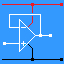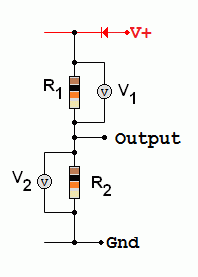RANDOM PAGE

SITE SEARCH

LOG
IN

HELP

# Voltage Dividers

This is the AQA version closing after June 2019. Visit the the version for Eduqas instead.R1 and R2 form a Voltage Divider. The voltage is divided in the ratio of the resistor values (assuming the current is the same in both resistors). Two or more resistors in series can be used to set a required voltage at a point in a circuit. For example, this could correspond to a threshold voltage at which an alarm should sound the voltage output required from a power supply circuit the volume needed from a radio the speed at which a fan runs. V2 = Vs R2 / (R1 + R2)    where Vs is the supply voltage. V1 = Vs R1/ (R1 + R2)    where Vs is the supply voltage. V1 / R1 = V2 / R2        or        V1 / V2 = R1 / R2        also        V1 + V2 = Vs

For the Falstad Circuit Simulation, CTRL+Click Voltage Divider Simulation
In options, check European Resistors and uncheck Conventional Current.
Click the switch to turn the circuit on or off. Watch the voltages change.

Alternatively view Voltage_Divider.txt.
Save or copy the text on the web page. Import the saved or copied text into the Falstad simulator.

Here is the new HTML5 Simulator Site.

reviseOmatic V3     Contacts, ©, Cookies, Data Protection and Disclaimers Hosted at linode.com, London